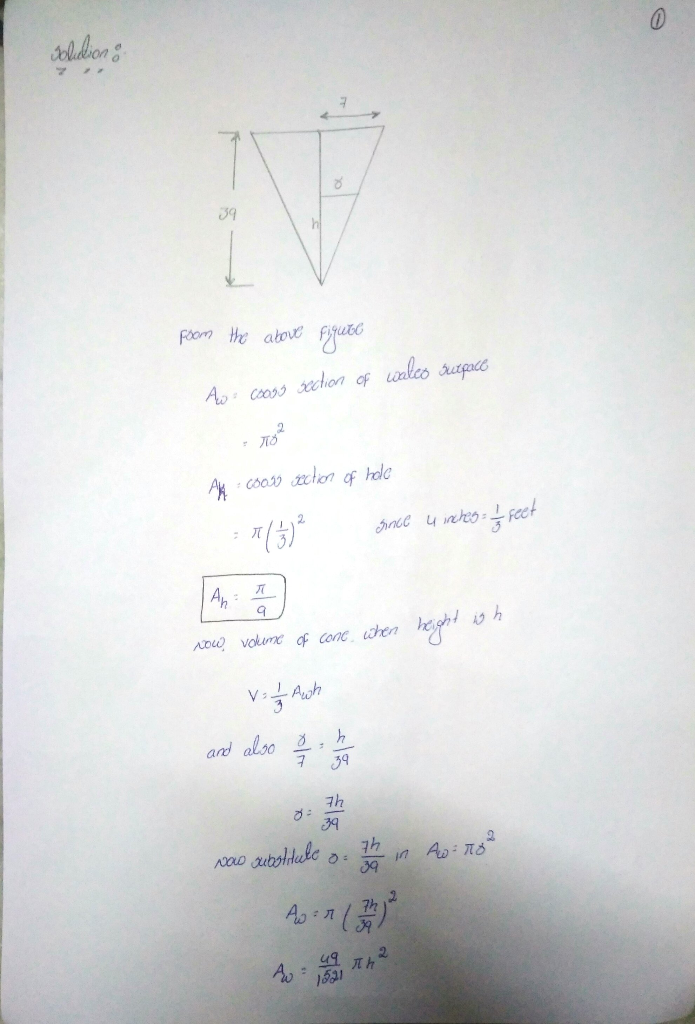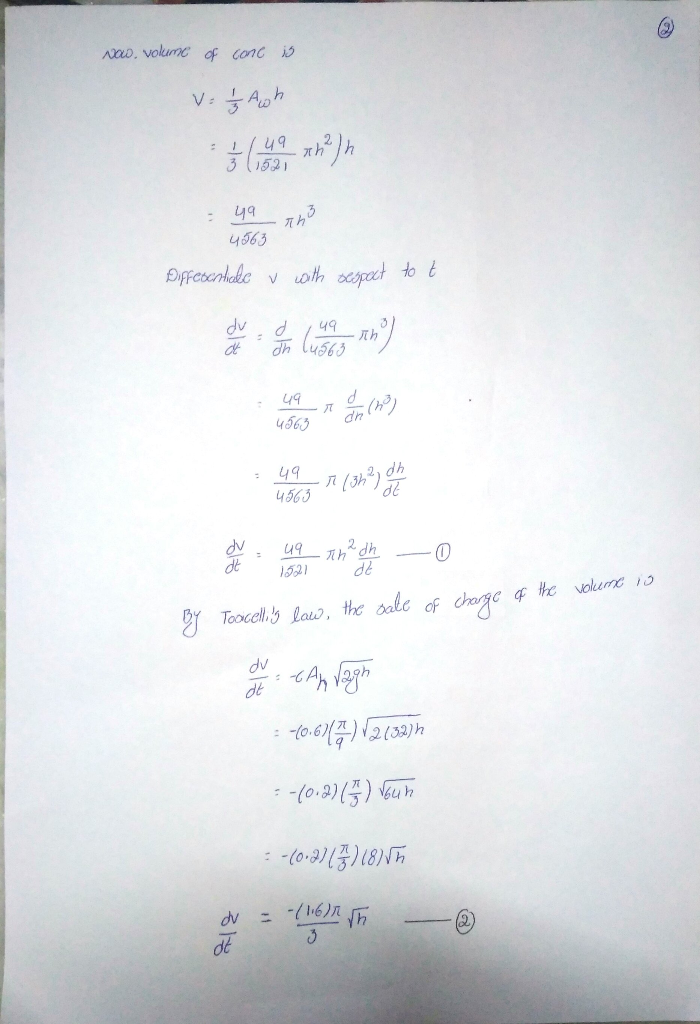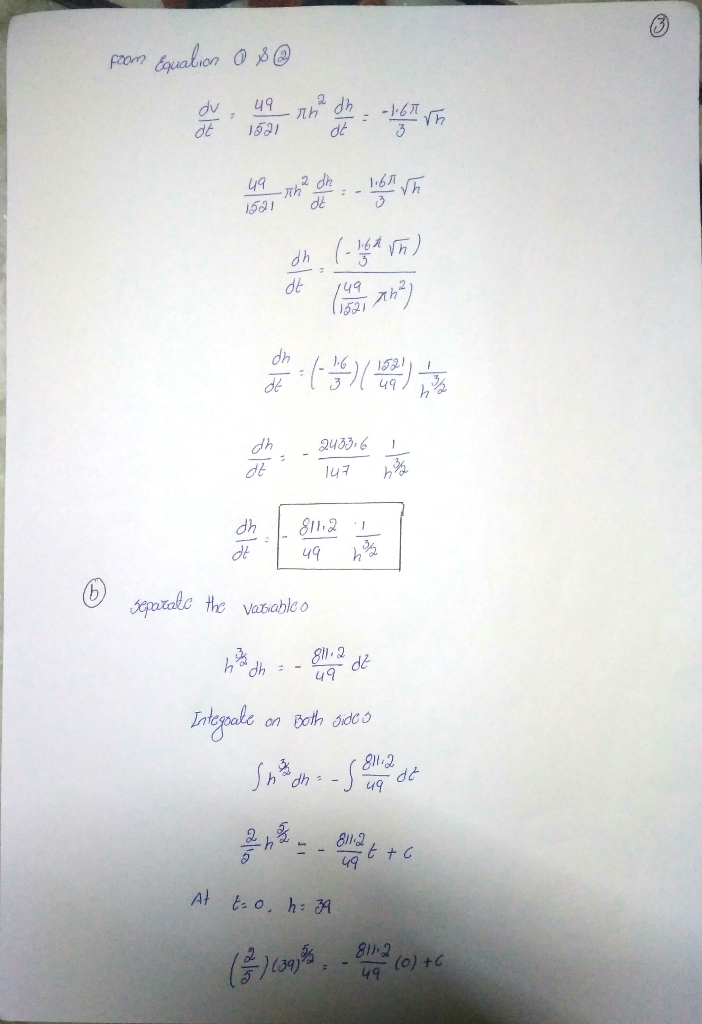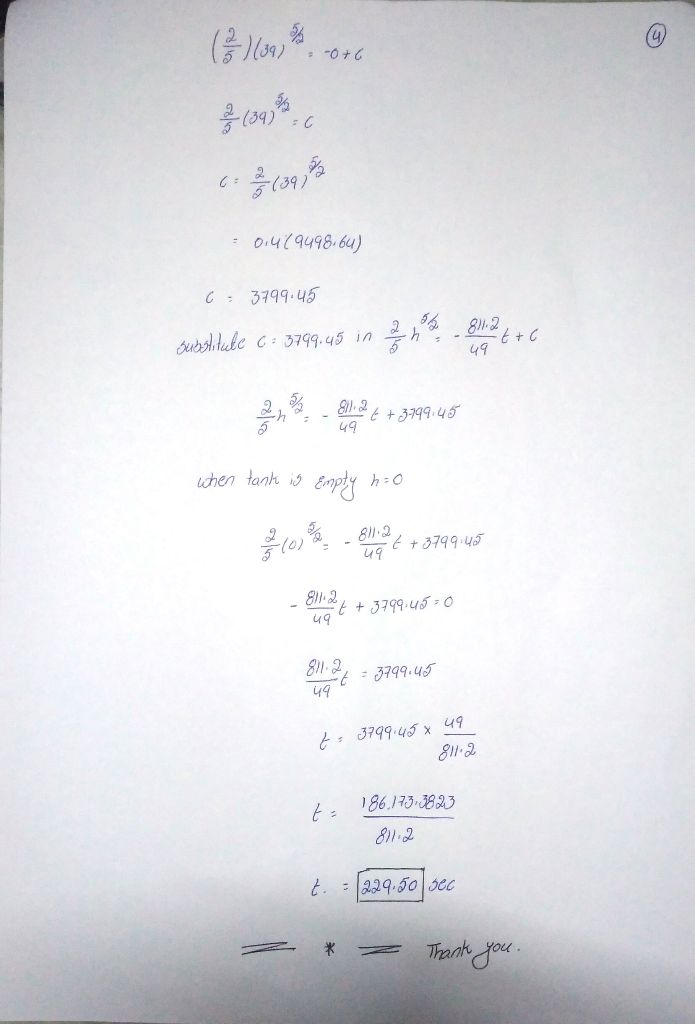# The tank in the form of a right-circular cone of radius 7 feet and height 39...

The tank in the form of a right-circular cone of radius 7 feet and height 39 feet standing on its end, vertex down, is leaking through a circular hole of radius 4 inches. Assume the friction coefficient to be c=0.6 and g=32ft/s^2 . Then the equation governing the height h of the leaking water is

dhdt=_______________

If the tank is initially full, it will take _________ seconds to empty.

## Solutions

##### Expert SolutionDear student if you have any doubt Please ask, Thank you.

## Related Solutions

##### The surface area of a right-circular cone of radius r and height h is S=πrr2+h2−−−−−−√, and...
The surface area of a right-circular cone of radius r and height h is S=πrr2+h2−−−−−−√, and its volume is V=1/3πr2h. (a) Determine h and r for the cone with given surface area S=4 and maximal volume V. h=  , r= (b) What is the ratio h/r for a cone with given volume V=4 and minimal surface area S? hr= (c) Does a cone with given volume V and maximal surface area exist? A. yes B. no
##### The volume of a right circular cylinder with base radius ? and height ℎ is given...
The volume of a right circular cylinder with base radius ? and height ℎ is given by: ? = ??^2ℎ. If the base radius is decreasing at a rate of 3 inches per minute and the height is increasing at a rate of 2 inches per minute, at what rate is the volume of the cylinder changing when the radius is 8 inches and the height is 3 inches. Will the volume be increasing or decreasing at this instant? Be...
##### Let K be a cone with a circular bottom, that has a radius r, and the...
Let K be a cone with a circular bottom, that has a radius r, and the apex is directly above the center of the bottom. Let h represent the height of the cone. Show that the surface area of the cone K without the bottom is equal to pi * r * sqrt(r^2 + h^2) . (Use that a sector that is given with angle θ in a circle with radius R has the area  (θ * R^2)/2
##### A cone has radius 4 and slant height 5. The cone is melted down and recast...
A cone has radius 4 and slant height 5. The cone is melted down and recast as a cylinder with height 4. The radius of the cylinder is? Show your work.
##### A water tank is spherical in shape with radius of 90 feet. Suppose the tank is...
A water tank is spherical in shape with radius of 90 feet. Suppose the tank is filled to a depth of 140 feet with water. Some of the water will be pumped out and, at the end, the depth of the remaining water must be 40 feet. i) Set up a Riemann sum that approximates the volume of the water that is pumped out of the tank. (use horizontal slicing). You have to draw a diagram and choose a coordinate...
##### Use Cavalieri’s principle to derive a formula for volume of a right circular cone.
Use Cavalieri’s principle to derive a formula for volume of a right circular cone.
##### In the figure current is set up through a truncated right circular cone of resistivity ?,...
In the figure current is set up through a truncated right circular cone of resistivity ?, left radius a, right radius b, and length L. Assume that the current density is uniform across any cross section taken perpendicular to the length. In terms of the variables given, what is the resistance of the cone?
##### An underground tank is a hemisphere with radius 4 feet (i.e. it is half a sphere,...
An underground tank is a hemisphere with radius 4 feet (i.e. it is half a sphere, and so horizontal cross-sections are circles of varying radius). It is filled to the top with oil weighing 45 lb per cubic foot. The top of the tank is at ground level, and the oil in the tank is pumped to a height of 10 feet above ground level. How much work is done? Set up the integral, but then just use your calculator...
##### An observatory is to be in the form of a right circular cylinder surmounted by a...
An observatory is to be in the form of a right circular cylinder surmounted by a hemispherical dome. If the hemispherical dome costs 10 times as much per square foot as the cylindrical​ wall, what are the most economic dimensions for a volume of 10000 cubic​ feet?
##### A spherical ball was completely immersed into an inverted right circular cone full of water. After...
A spherical ball was completely immersed into an inverted right circular cone full of water. After the ball was removed it was found out that the water surface had dropped 6 cm. below the top of the cone. The diameter of the cone is 12 cm. and its altitude is 36 cm. Determine the wet area (in cm^2) when the cone is full.